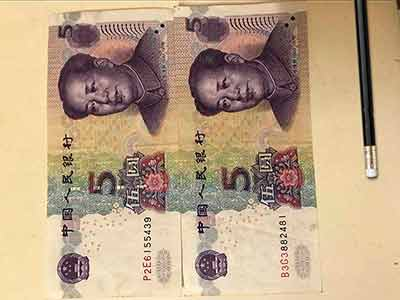# FRM二级精选题型解析：A portfolio manager determines

A portfolio manager determines that his portfolio has an expected return of \$20,000 and a standard deviation of \$45,000.

Given a 95 percent confidence level, what is the portfolio's VAR?

A) \$43,500.

B) \$74,250.

C) \$94,250.

D) \$54,250.D was incorrect.

The expected outcome is \$20,000. Given the standard deviation of \$45,000 and a z-score of 1.65 (95% confidence level for a one-tailed test), the VAR is –54,250 [=20,000 – 1.65 (45,000)].